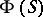# Semi-group algebra

(diff) ← Older revision | Latest revision (diff) | Newer revision → (diff)
An algebraover a fieldwith a basisthat is at the same time a multiplicative semi-group. In particular, ifis a group, one obtains a group algebra. If the semi-groupcontains a zero, this zero is usually identified with the zero of the algebra. The problem of describing all linear representations of a semi-groupover a field(cf. Linear representation; Representation of a semi-group) is equivalent to that of describing all representations of the algebra. The importance of semi-group algebras in the theory of semi-groups is the possibility they offer of utilizing the richer tools of the theory of algebras to study linear representations of semi-groups. An example of this kind of result is: The algebraof a finite semi-groupis semi-simple if and only if all linear representations of the semi-groupover the algebraare reducible.